# Two Pointer Interview Questions & Tips

By The Mighty Anomaly | Published: July 12, 2023

## What Is the Two Pointer Technique?

The two-pointer technique is a pattern where two pointers iterate over the data structure in tandem or separately until they satisfy a certain condition. This pattern is extremely useful when dealing with linear data structures such as arrays, linked lists, or strings.

Two pointer problems are among the most common types you will encounter during coding interviews. This technique involves using two pointers which move through the array or list in a synchronized or independent manner to solve a problem. The two pointer technique is a flexible and versatile strategy that can be applied to various scenarios, such as finding pairs in a sorted array, checking for a palindrome, or finding a cycle in a linked list. Two-pointer algorithms generally rely on pointer manipulation (in this context a “pointer” is just an index in an array or similar linear data structure and does not refer to memory addresses of objects like pointers in C-based contexts do).

### Two Pointer Types

#### Polynomial Pointers (for brute force combinations)

This pointer variety is generally considered the least beneficial, as it often necessitates the use of nested loops. Each pointer, in this setup, travels independently across each index of the input. As a result, this pattern typically results in a polynomial (commonly quadratic – `O(N^2)`) time complexity due to the fact that it computes all potential combinations of two pointers within a single linear input. The bubble sort algorithm exemplifies this kind of pointer usage.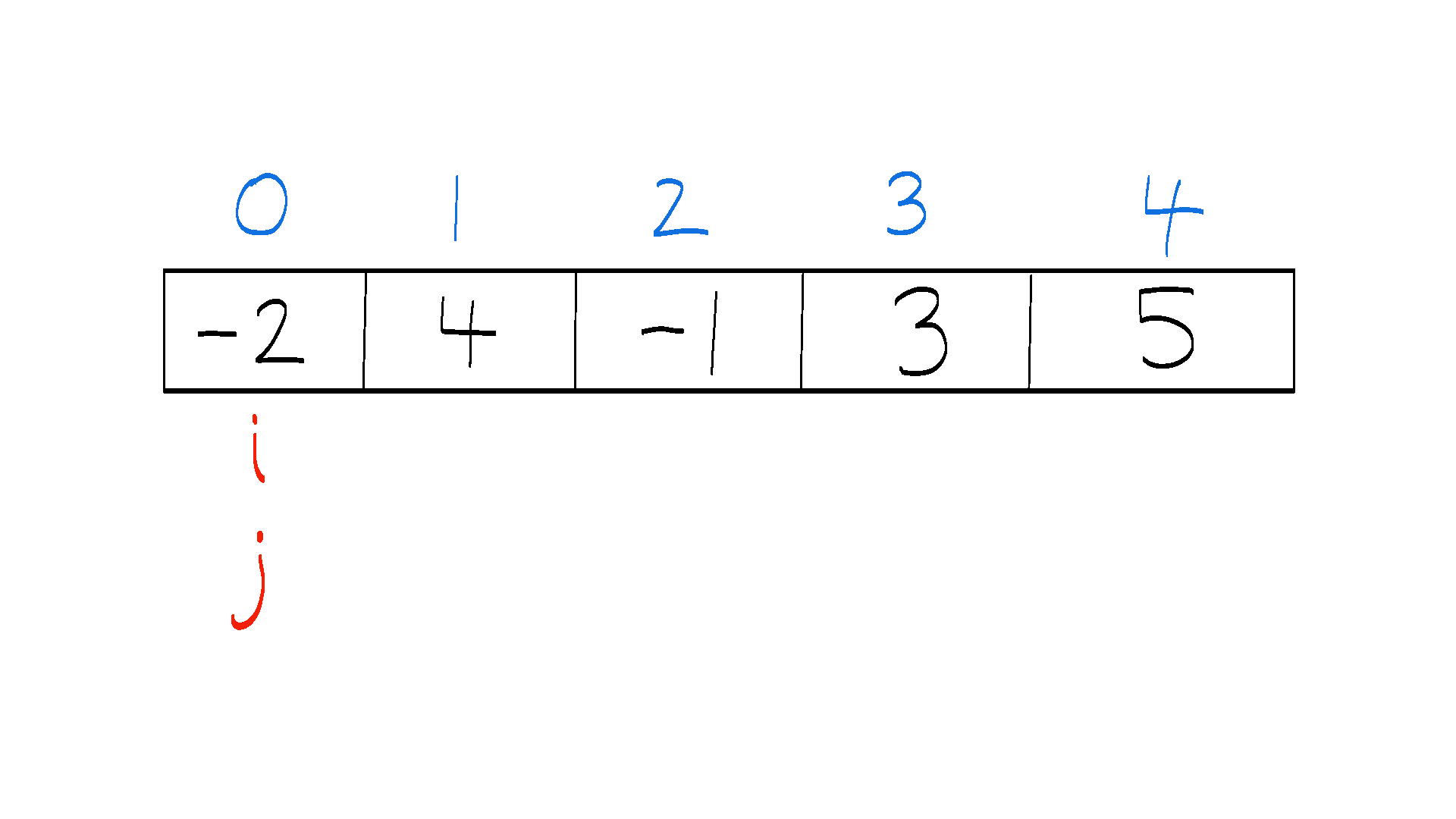Generate Subarrays: Generate all subarrays of the given array input

#### Pointer Chasing ("Slow and Fast" or "Tortoise and Hare") for Cycle Detection

This variant of the two-pointer technique is notably applied in identifying cycles in linked lists and graphs, as demonstrated in Floyd's algorithm. In this setup, both pointers initiate their journey from the beginning of the input (typically index 0) but progress at varied speeds. Generally, the "tortoise" pointer advances by just one index in each step, whereas the "hare" pointer leaps forward by two indices at a time.Floyd's Cycle Detection: Given the head of a Linked List, determine if a cycle exists in the Linked List

#### Rivaling Pointers

In this variant of the two-pointer technique, one pointer commences at the start of the input, while the other initiates from the input's end. These pointers subsequently shift inwardly (toward each other) alternately, adhering to a predefined logic. The binary search algorithm or Palindrome problem both serve as textbook examples of this type of problem.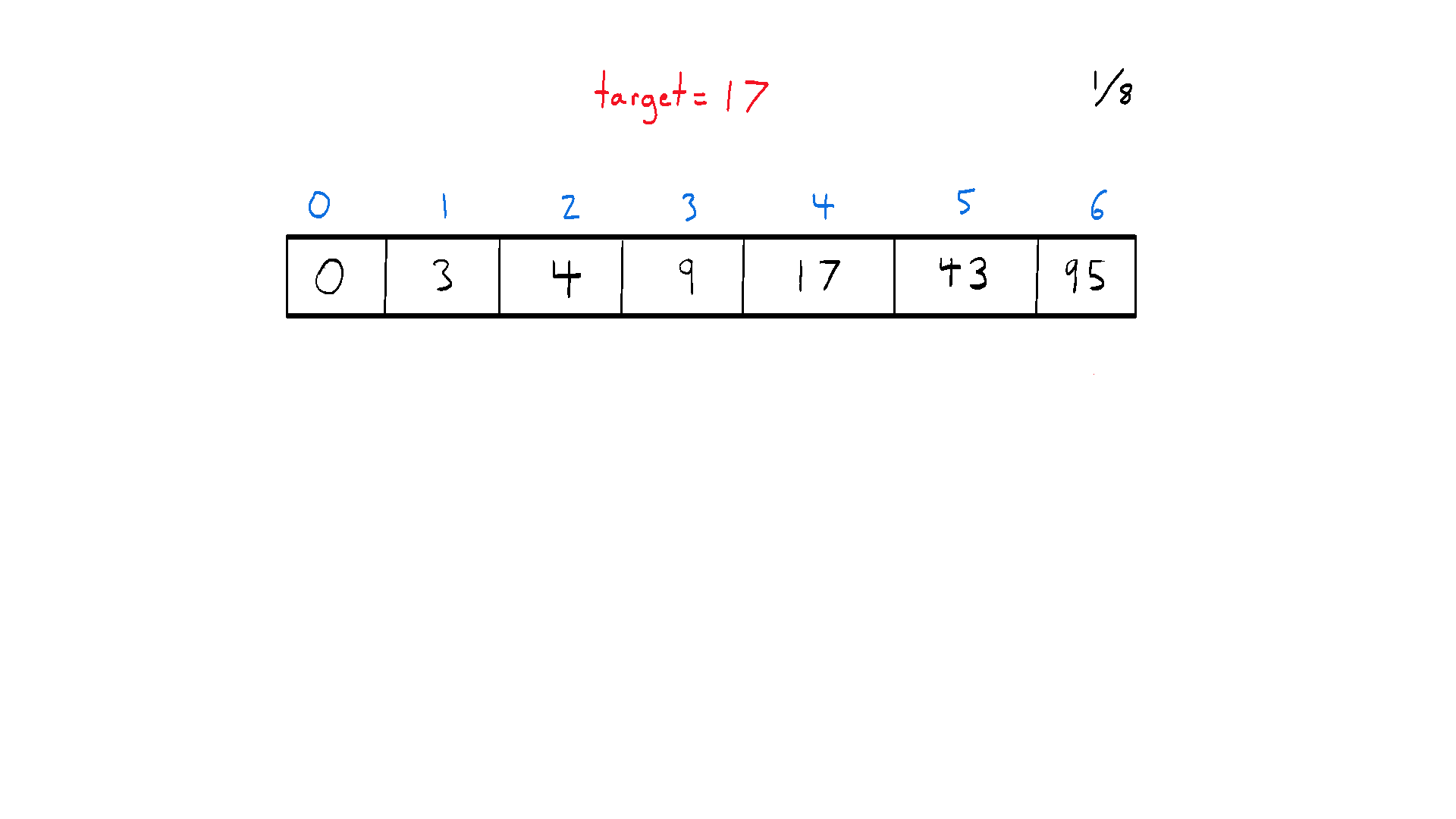Binary Search: Given a sorted array and a target, determine if the element exists in the array

#### Multiple Pointers

While traditionally two-pointer problems involve exactly two pointers, it's not uncommon to encounter problems where more than two pointers can be beneficial. The "Multiple Pointers" technique typically involves more than two pointers that move independently or in a correlated manner based on specific logic.

This type of problem often shows up in more complex array or string problems, where the interaction between multiple elements at different positions needs to be considered. For instance, a problem might require you to sort an array of zeros, ones, and twos as in the Dutch Flag problem. A polynomial pointer approach can solve the problem in quadratic time. However, by cleverly segregating the array with three pointers, you can reduce the time complexity to linear time.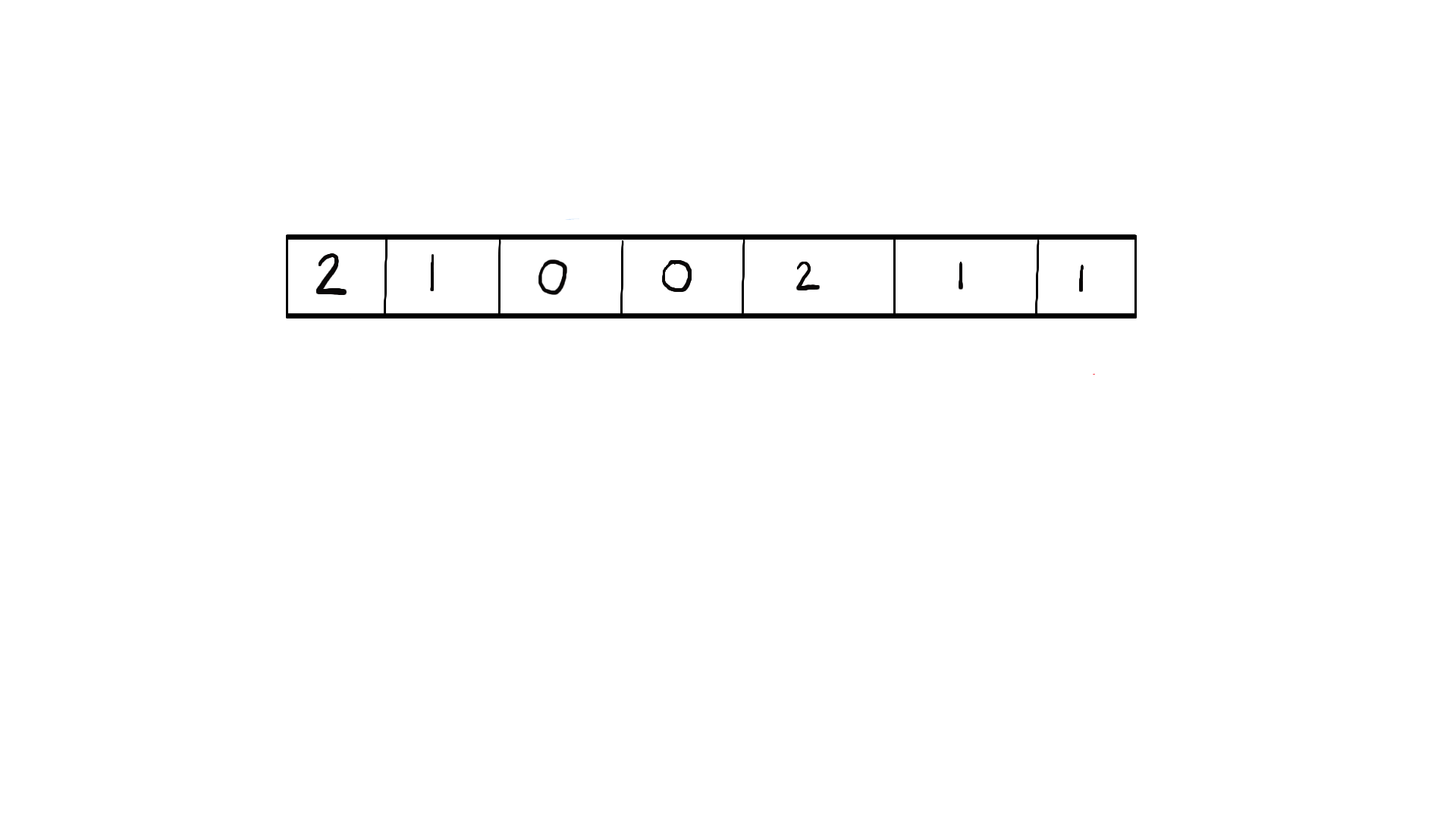Dutch Flag: Multiple pointer approach to sorting 0's, 1's, and 2's.

#### Double Input Pointers

This pointer type is interesting since it involves two linear inputs with a single pointer on each, rather than a single input with two pointers. A common example of this would be the “merge” part of the Merge Sort algorithm in which we merge two sorted lists into a single list by carefully comparing and updating pointers in each list based on their relative values.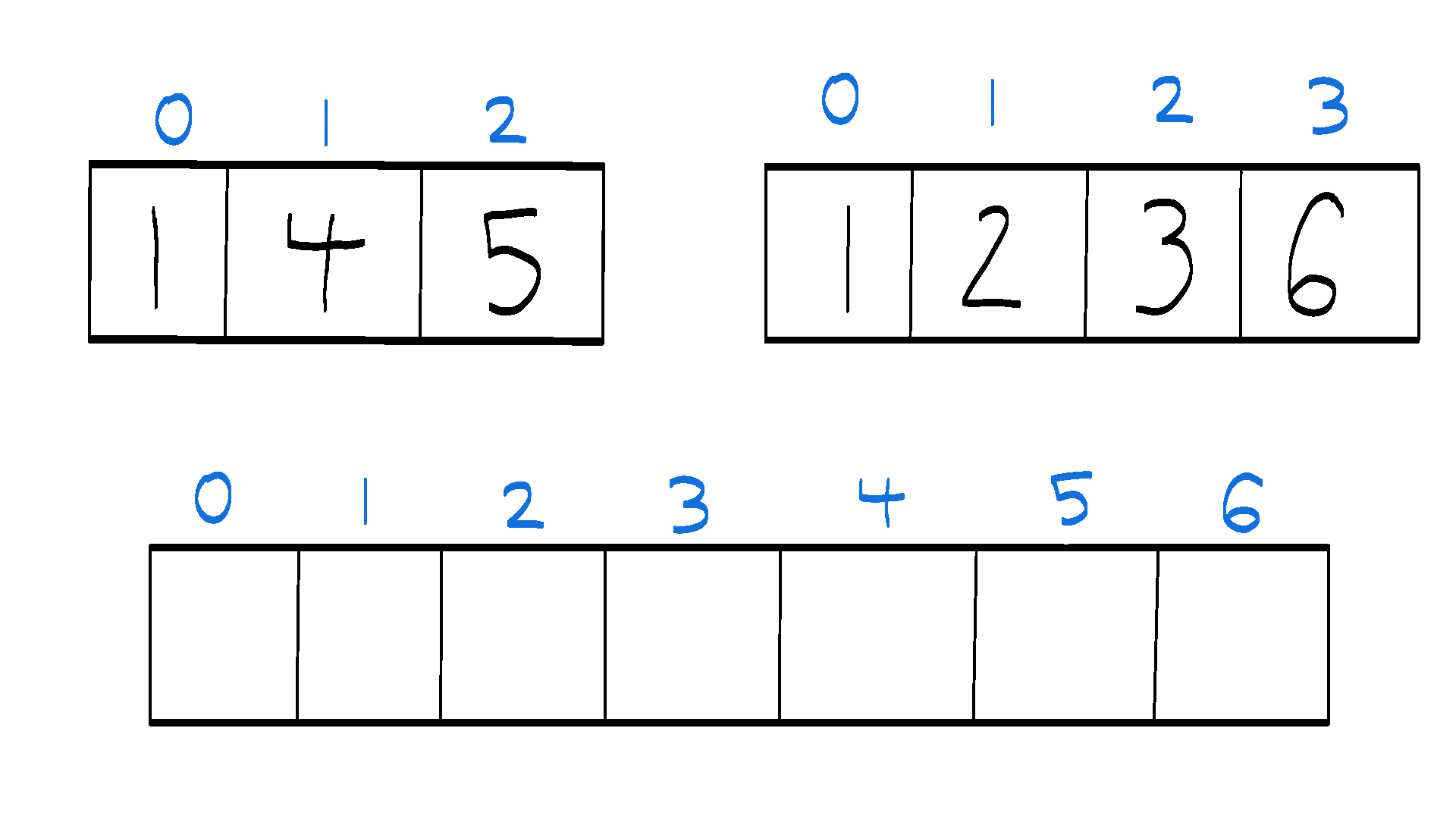Merging Two Sorted Lists: Algorithm merges two sorted lists together in linear time

#### Sliding Windows

So much can be said about this two pointer problem subtype that we have a whole separate guide on it! Predominantly observed in array and string questions, this variety of two-pointer problem aids in locating subarrays or substrings within an input. It is crucial to acknowledge that subarrays and substrings represent continuous segments of a linear input. This distinction is significant since we exploit the contiguous characteristic of a substring to bypass redundant computations from previous steps and avoid the typical polynomial pointers (brute force) approach.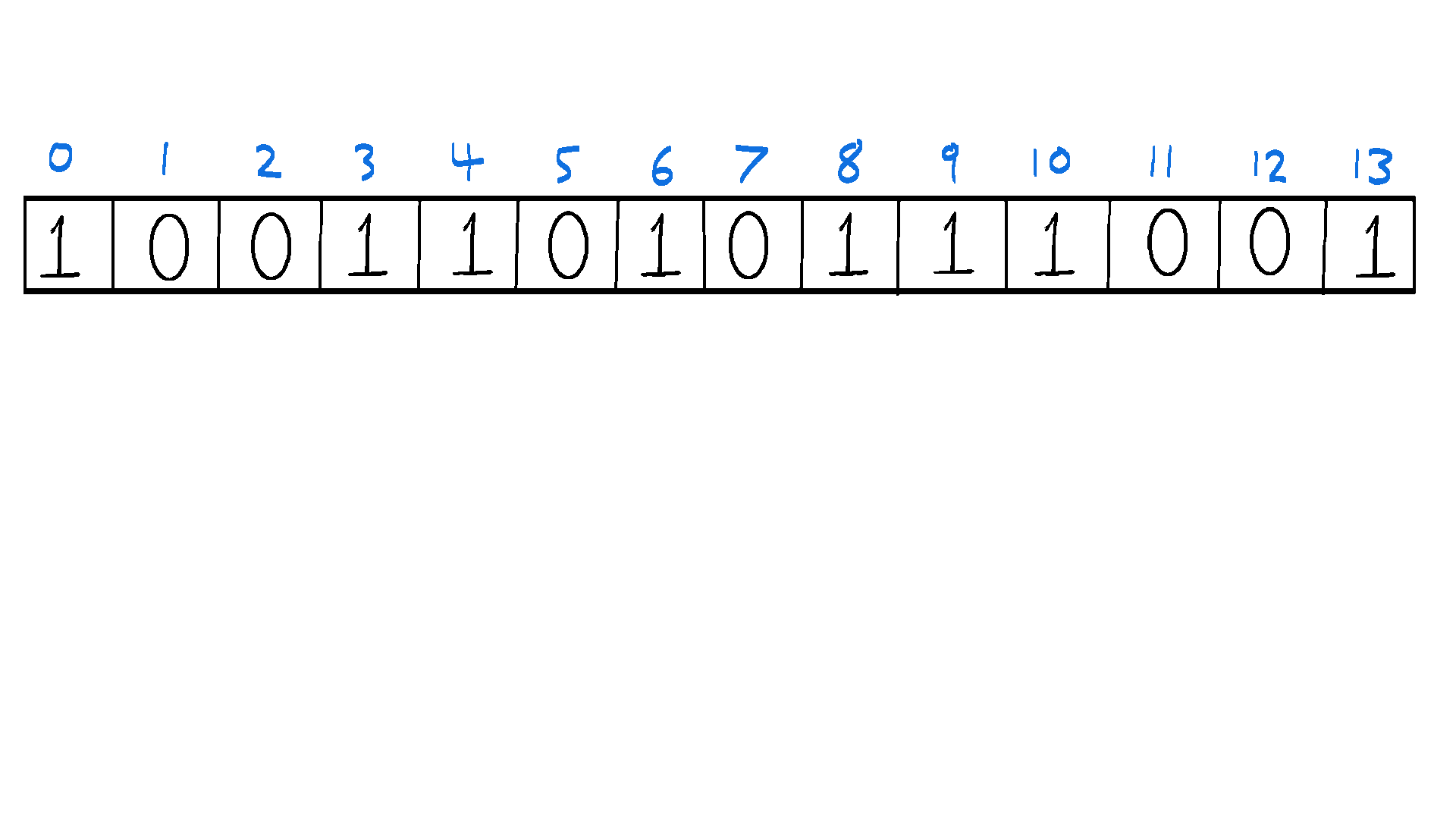Fixed Window Pattern: Given an array of 0's and 1's and a number k, find the subarray of length k with the most 1's (k = 5 in the example above)

## When to Use Two Pointers in Interviews

Two pointer problems usually surface when dealing with ordered data or when there is a need to eliminate redundant computations. Similar to the above, here are a few scenarios when this technique can be particularly handy:

• Searching pairs in a sorted array: The pointers can start at both ends of the array and move inward until they meet, eliminating possible pairs along the way. This method is faster than using a nested loop to check all pairs.
• Finding a cycle in a linked list: One pointer can move faster (2 steps at a time), and the other slower (1 step at a time). If there is a cycle, the faster pointer will eventually meet the slower one.
• Checking for palindromes: The pointers can start at both ends and move inward, checking if the mirrored characters are the same.
• Applying a sliding window: One pointer marks the start of the window, the other the end. The window can then be 'slid' through the array to check for conditions.

### Valid Palindrome Example

#### Problem Statement

``````Given a string s, determine if it is a palindrome, considering only
alphanumeric characters and ignoring cases.
``````

#### Intuition

The two-pointer technique is highly effective for this problem. We can initiate two pointers, one at the start and one at the end of the string. We then move the pointers towards the center of the string. At each step, we check if the characters at the two pointers are the same. If not, the string is not a palindrome. We can ignore non-alphanumeric characters by advancing the corresponding pointer(s) until we reach an alphanumeric character. We also need to ensure our comparison is case-insensitive.

#### Code

``````def isPalindrome(s):
l, r = 0, len(s) - 1
while l < r:
if not s[l].isalnum():
l += 1
elif not s[r].isalnum():
r -= 1
elif s[l].lower() == s[r].lower():
l += 1
r -= 1
else:
return False
return True
``````

### Two Sum II Example

#### Problem Statement

``````Given a 1-indexed array of integers numbers that is already sorted in non-decreasing order,
find two numbers such that they add up to a specific target number. Let these two numbers
be numbers[i] and numbers[j] where 1 <= i < j <= numbers.length.
``````

#### Intuition

The two-pointer technique works well here because we can start with a pointer at each end of the sorted array. Since the array is sorted, if the sum of the two numbers pointed by the two pointers is less than the target, we can move the left pointer to the right to make the sum larger. Conversely, if the sum is larger than the target, we can move the right pointer to the left to make the sum smaller. This way, we can find the two numbers that add up to the target in linear time.

#### Code

``````def twoSum(nums, target):
l, r = 0, len(nums) - 1
while l < r:
current_sum = nums[l] + nums[r]
if current_sum == target:
return [l + 1, r + 1]
elif current_sum < target:
l += 1
else:
r -= 1
``````

The above problem and solution illustrate how the two-pointer technique helps solve problems efficiently. This strategy is a useful tool to have in your programming arsenal, especially for interview preparation. Understanding the fundamental concepts behind this technique and practicing it on problems like those above can give you a solid foundation for tackling similar problems.

## Common Mistakes in Interviews Featuring Two Pointers

Here are common mistakes to avoid when tackling two pointer problems in interviews, note that almost all of these involve Index Out Of Bounds errors if not planned for:

• Not initializing pointers correctly: The initialization of pointers greatly depends on the problem at hand. Ensure you understand the problem statement thoroughly before deciding where to place the pointers initially.
• Not moving pointers correctly: Again, the movement of the pointers depends on the problem. In some problems, both pointers move together, while in others, they may move independently.
• Not handling edge cases: Be careful with edge cases. For instance, what if the array is empty? Or what if the array has only one element? Make sure your solution handles these scenarios gracefully.
• Forgetting to update pointers or conditions: This can lead to an infinite loop, especially when dealing with while-loops. Always double-check your code to ensure pointers are updated correctly inside the loop.

## What to Say in Interviews to Show Mastery Over Two Pointers

Two pointer questions are an odd question type since they tend to be simple to explain, but deceptively complex to implement bug-free. Here are a few suggested phrases that demonstrate your understanding of the two-pointer technique:

• Recognize inputs that help you: "Given the sorted nature of the input, we can use a two-pointer technique to eliminate pairs and potentially reduce the time complexity from `O(N^2)` to `O(N)`."
• Understand the sliding window technique: "We can employ the two-pointer technique here to create a sliding window, which can help us maintain a running total within the window size, and update it as the window slides through the array."
• Show awareness of cycle detection algorithms: "We can use the two-pointer technique to traverse this linked list. One pointer will go two steps at a time while the other moves one step at a time. If there's a cycle, the fast pointer will eventually catch up with the slow pointer."

Before jumping into solving two-pointer problems, it's a good idea to ask the following questions to get a better understanding of the problem:

• Clarify the target complexity: "Are there any constraints on the space complexity of the solution? Can we use additional data structures?"
• Clarify potentially unexpected input examples: "Can the input array or list contain duplicates?"
• Clarify what to do if no answer is possible: "What should the function return if no solution is found?"
• Make no assumptions: "I see the input is sorted in this example, but can we always assume the input data sorted? If it's possible we get unsorted data, are we allowed to mutate it with an in-place sort?"

## Two Pointer Frequently Asked Questions (FAQ)

Here are some frequently asked questions and their answers regarding the two-pointer technique:

### Can We Use More Than Two Pointers in a Problem?

Yes, it's possible to use more than two pointers. The 'two-pointer' term is more of a general idea than a strict rule. Depending on the complexity of the problem, we may need more pointers.

## How Is Binary Search a Two-Pointer Type?

In a binary search, two pointers (usually referred to as 'left and 'right') are used to keep track of the remaining search space. After each step, the search space is halved by moving either the 'left' or 'right' pointer, hence the name 'binary'.

## How Is the Sliding Window a Two-Pointer Type?

In sliding window problems, we maintain a 'window' of elements in an array or list using two pointers, where one pointer represents the start of the window and the other the end of the window. As we move through the array, we 'slide' this window by moving both pointers.

## Common Two-Pointer interview QuestionsThe Mighty Anomaly

The Mighty Anomaly (Mike) is one of the top Google coaches for interviewing.io having personally helped over 100+ engineers get into Google with his unique coaching style. After receiving offers from the full FAANG gauntlet early in his career, he enjoys teaching others proven techniques to pass technical interviews with his decade of coaching and industry experience.